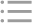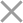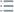## Fill out the form in 2 simple steps below:

Step 1

Step 2
** By clicking this button you agree to our Privacy Policy statementRequest our prices
--Select currency--
USD
EUR
* By clicking this button you agree to our Privacy Policy statementFree PVS‑Studio license for Microsoft MVP specialists
* By clicking this button you agree to our Privacy Policy statementTo get the licence for your open-source project, please fill out this form
* By clicking this button you agree to our Privacy Policy statementI am interested to try it on the platforms:
 Windows Linux macOS PVS-Studio for .NET Core JetBrains Rider
* By clicking this button you agree to our Privacy Policy statementMessage submitted.

Your message has been sent. We will email you at

check your Spam/Junk folder and click the "Not Spam" button for our message.
This way, you won't miss messages from our team in the future.

>
>
>
V101. Implicit assignment type conversi…Introduction
Analyzing projects
On Windows
On Linux and macOS
Continuous use of the analyzer in software development
Deploying the analyzer in cloud Continuous Integration services
Managing analysis results
Analyzer diagnostics
General Analysis (C++)
General Analysis (C#)
General Analysis (Java)
Diagnosis of micro-optimizations (C++)
Diagnosis of micro-optimizations (C#)
Diagnosis of 64-bit errors (Viva64, C++)
Customer specific requests (C++)
MISRA errors
AUTOSAR errors
OWASP errors (C++)
OWASP errors (C#)
OWASP errors (Java)
Problems related to code analyzerContents

# V101. Implicit assignment type conversion to memsize type.

Jun 13 2012

The analyzer detected a potential error relating to implicit type conversion while executing the assignment operator "=". The error may consist in incorrect calculating of the value of the expression to the right of the assignment operator "=".

An example of the code causing the warning message:

``````size_t a;
unsigned b;
...
a = b; // V101``````

The operation of converting a 32-bit type to memsize-type is safe in itself as there is no data loss. For example, you can always save the value of unsigned-type variable into a variable of size_t type. But the presence of this type conversion may indicate a hidden error made before.

The first cause of the error occurrence on a 64-bit system may be the change of the expression calculation process. Let's consider an example:

``````unsigned a = 10;
int b = -11;
ptrdiff_t c = a + b; //V101
cout << c << endl;``````

On a 32-bit system this code will display the value -1, while on a 64-bit system it will be 4294967295. This behaviour fully meets the rules of type converion in C++ but most likely it will cause an error in a real code.

Let's explain the example. According to C++ rules a+b expression has unsigned type and contains the value 0xFFFFFFFFu. On a 32-bit system ptrdiff_t type is a sign 32-bit type. After 0xFFFFFFFFu value is assigned to the 32-bit sign variable it will contain the value -1. On a 64-bit system ptrdiff_t type is a sign 64-bit type. It means 0xFFFFFFFFu value will be represented as it is. That is, the value of the variable after assignment will be 4294967295.

The error may be corrected by excluding mixed use of memsize and non-memsize-types in one expression. An example of code correction:

``````size_t a = 10;
ptrdiff_t b = -11;
ptrdiff_t c = a + b;
cout << c << endl;``````

A more proper way of correction is to refuse using sign and non-sign data types together.

The second cause of the error may be an overflow occurring in 32-bit data types. In this case the error may stand before the assignment operator but you can detect it only indirectly. Such errors occur in code allocating large memory sizes. Let's consider an example:

``````unsigned Width  = 1800;
unsigned Height = 1800;
unsigned Depth  = 1800;
// Real error is here
unsigned CellCount = Width * Height * Depth;
// Here we get a diagnostic message V101
size_t ArraySize = CellCount * sizeof(char);
cout << ArraySize << endl;
void *Array = malloc(ArraySize);``````

Suppose that we decided to process data arrays of more than 4 Gb on a 64-bit system. In this case the given code will cause allocation of a wrong memory size. The programmer is planning to allocate 5832000000 memory bytes but he gets only 1537032704 instead. It happens because of an overflow occurring while calculating Width * Height * Depth expression. Unfortunately, we cannot diagnose the error in the line containing this expression but we can indirectly indicate the presence of the error detecting type conversion in the line:

``size_t ArraySize = CellCount * sizeof(char); //V101``

To correct the error you should use types allowing you to store the necessary range of values. Mind that correction of the following kind is not appropriate:

``size_t CellCount = Width * Height * Depth;``

We still have the overflow here. Let's consider two examples of proper code correction:

``````// 1)
unsigned Width  = 1800;
unsigned Height = 1800;
unsigned Depth  = 1800;
size_t CellCount =
static_cast<size_t>(Width) *
static_cast<size_t>(Height) *
static_cast<size_t>(Depth);
// 2)
size_t Width  = 1800;
size_t Height = 1800;
size_t Depth  = 1800;
size_t CellCount = Width * Height * Depth;``````

You should keep in mind that the error can be situated not only higher but even in another module. Let's give a corresponding example. Here the error consists in incorrect index calculation when the array's size exceeds 4 Gb.

Suppose that the application uses a large one-dimensional array and CalcIndex function allows you to address this array as a two-dimensional one.

``````extern unsigned ArrayWidth;
unsigned CalcIndex(unsigned x, unsigned y) {
return x + y * ArrayWidth;
}
...
const size_t index = CalcIndex(x, y); //V101``````

The analyzer will warn about the problem in the line: const size_t index = CalcIndex(x, y). But the error is in incorrect implementation of CalcIndex function. If we take CalcIndex separately it is absolutely correct. The output and input values have unsigned type. Calculations are also performed only with unsigned types participating. There are no explicit or implicit type conversions and the analyzer has no opportunity to detect a logic problem relating to CalcIndex function. The error consists in that the result returned by the function and possibly the result of the input values was chosen incorrectly. The function's result must have memsize type.

Fortunately, the analyzer managed to detect implicit conversion of CalcIndex function's result to size_t type. It allows you to analyze the situation and bring necessary changes into the program. Correction of the error may be, for example, the following:

``````extern size_t ArrayWidth;
size_t CalcIndex(size_t x, size_t y) {
return x + y * ArrayWidth;
}
...
const size_t index = CalcIndex(x, y);``````

If you are sure that the code is correct and the array's size will never reach 4 Gb you can suppress the analyzer's warning message by explicit type conversion:

``````extern unsigned ArrayWidth;
unsigned CalcIndex(unsigned x, unsigned y) {
return x + y * ArrayWidth;
}
...
const size_t index = static_cast<size_t>(CalcIndex(x, y));``````

In some cases the analyzer can understand itself that an overflow is impossible and the message won't be displayed.

Let's consider the last example related to incorrect shift operations

``````ptrdiff_t SetBitN(ptrdiff_t value, unsigned bitNum) {
ptrdiff_t mask = 1 << bitNum; //V101